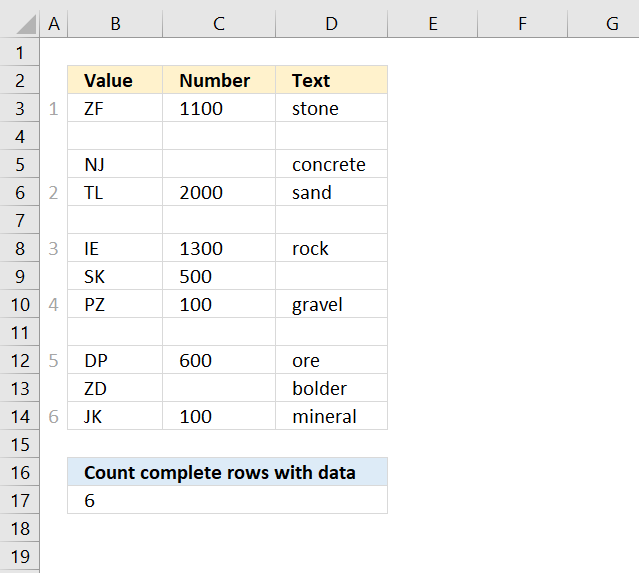Author: Oscar Cronquist Article last updated on October 31, 2018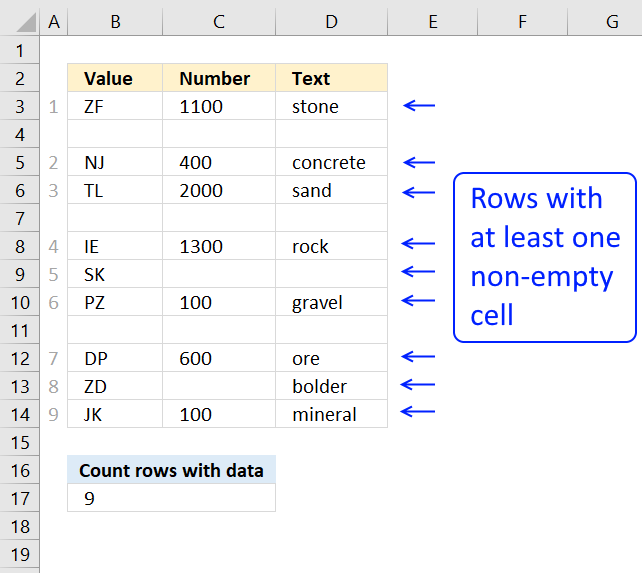The formula in cell B17 counts rows in cell range B3:D17 when at least one cell per row contains data.

Formula in cell B17:

=SUMPRODUCT((MMULT((B3:D14="")*1,{1;1;1})<3)*1)

If your data set, for example, has 5 columns change:

• B3:D14 to your cell range
• the array from {1;1;1} to {1;1;1;1;1}, there must be as many 1's as there a columns in your data set.
• also change <3 to <5

Example, your cell range is A3:G14. The formula becomes:

=SUMPRODUCT((MMULT((A3:G14="")*1,{1;1;1;1;1;1;1})<7)*1)

### Explaining formula in cell B17

Step 1 - Check if cell is empty

The equal sign allows you to compare each cell in B3:D14 with an empty value "".

B3:D14="" returns an array of boolean values indicating if a cell is empty or not. {FALSE, FALSE, FALSE; ... }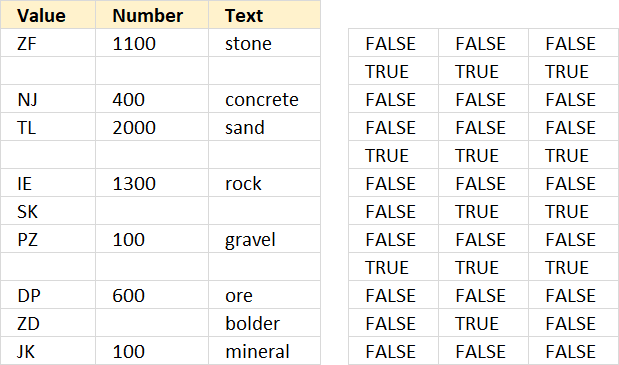The picture above shows the array to the right and the corresponding values to the left.

Step 2 - Convert boolean values to numbers

To convert the boolean array to 1 and 0 (zero) I multiply with 1. The parentheses allow you to determine the order of operation.

I want to compare the values with "" before I mutlitply with 1.

(B3:D14="")*1 returns {0, 0, 0; ...)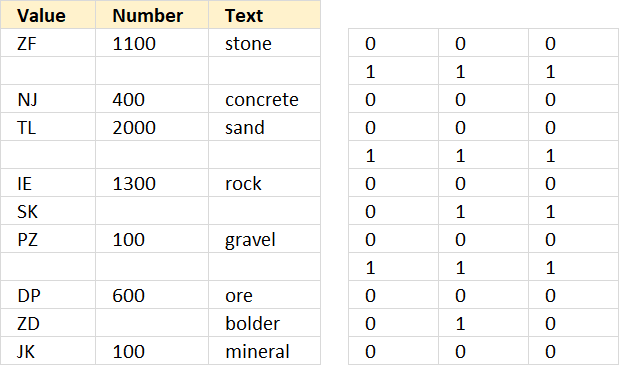The picture above shows the array to the right.

Step 3 - Add values row-wise

The MMULT function is great for adding values row by row, however, it can not handle boolean values. The function returns an array of values.

MMULT((B3:D14="")*1,{1;1;1})

There are two arguments in the MMULT function, array1 and array2.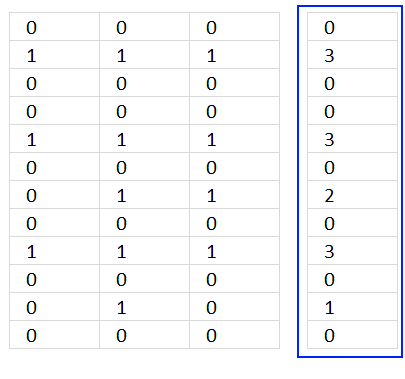The picture above shows you the result from the MMULT function in the blue rectangle.

How to use the MMULT function

The MMULT function calculates the matrix product of two arrays, an array as the same number of rows as array1 and […]

Step 4 - Check if each value in the array is smaller than 3.

If there are three empty values in a row that row is empty. That is why I check if each row is less than 3 indicating that at least one cell is not empty.

MMULT((B3:D14="")*1,{1;1;1})<3 returns {TRUE; FALSE; TRUE; TRUE; FALSE; TRUE; TRUE; TRUE; FALSE; TRUE; TRUE; TRUE}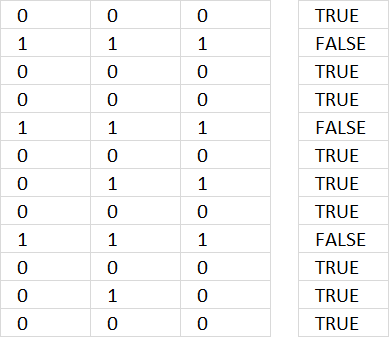The array is shown to the right in the picture above.

Step 5 - Count rows

To be able to sum the array of boolean values I have to multiply with 1 to convert them to 1 or 0 (zero). TRUE = 1 and FALSE = 0.

SUMPRODUCT((MMULT((A3:G14="")*1,{1;1;1;1;1;1;1})<7)*1)

becomes

SUMPRODUCT({TRUE; FALSE; TRUE; TRUE; FALSE; TRUE; TRUE; TRUE; FALSE; TRUE; TRUE; TRUE}*1)

becomes

SUMPRODUCT({TRUE; FALSE; TRUE; TRUE; FALSE; TRUE; TRUE; TRUE; FALSE; TRUE; TRUE; TRUE}*1)

becomes

SUMPRODUCT({1; 0; 1; 1; 0; 1; 1; 1; 0; 1; 1; 1}) and returns 9 in cell B17.

Why not use the SUM function? Then you would have to enter the formula as an array formula.